## 13.2.2 Linear Systems

Now that the phase space has been defined as a special kind of state space that can handle dynamics, it is convenient to classify the kinds of differential models that can be defined based on their mathematical form. The class of linear systems has been most widely studied, particularly in the context of control theory. The reason is that many powerful techniques from linear algebra can be applied to yield good control laws . The ideas can also be generalized to linear systems that involve optimality criteria [28,570], nature [95,564], or multiple players .

Let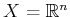be a phase space, and let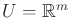be an action space for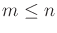. A linear system is a differential model for which the state transition equation can be expressed as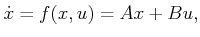(13.37)

in which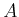and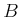are constant, real-valued matrices of dimensions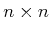and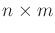, respectively.

Example 13..5 (Linear System Example)   For a simple example of (13.37), suppose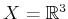,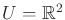, and let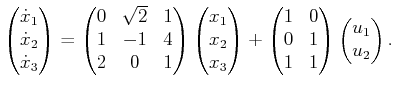(13.38)

Performing the matrix multiplications reveals that all three equations are linear in the state and action variables. Compare this to the discrete-time linear Gaussian system shown in Example 11.25.Recall from Section 13.1.1 thatlinear constraints restrict the velocity to an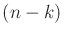-dimensional hyperplane. The linear model in (13.37) is in parametric form, which means that each action variable may allow an independent degree of freedom. In this case,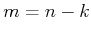. In the extreme case of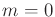, there are no actions, which results in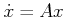. The phase velocity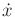is fixed for every point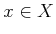. If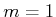, then at everya one-dimensional set of velocities may be chosen using. Note that the direction is not fixed because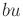is added to all components of. In general, the set of allowable velocities at a point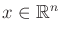is an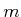-dimensional hyperplane in the tangent space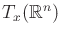(ifis nonsingular).

In spite of (13.37), it may still be possible to reach all of the state space from any initial state. It may be costly, however, to reach a nearby point because of the restriction on the tangent space; it is impossible to command a velocity in some directions. For the case of nonlinear systems, it is sometimes possible to quickly reach any point in a small neighborhood of a state, while remaining in a small region around the state. Such issues fall under the general topic of controllability, which will be covered in Sections 15.1.3 and 15.4.3.

Although not covered here, the observability of the system is an important topic in control [192,478]. In terms of the I-space concepts of Chapter 11, this means that a sensor of the form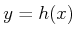is defined, and the task is to determine the current state, given the history I-state. If the system is observable, this means that the nondeterministic I-state is a single point. Otherwise, the system may only be partially observable. In the case of linear systems, if the sensing model is also linear,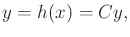(13.39)

then simple matrix conditions can be used to determine whether the system is observable . Nonlinear observability theory also exists .

As in the case of discrete planning problems, it is possible to define differential models that depend on time. In the discrete case, this involves a dependency on stages. For the continuous-stage case, a time-varying linear system is defined as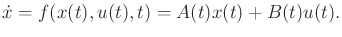(13.40)

In this case, the matrix entries are allowed to be functions of time. Many powerful control techniques can be easily adapted to this case, but it will not be considered here because most planning problems are time-invariant (or stationary).

Steven M LaValle 2020-08-14